cancel
Showing results for
Did you mean:Helper I

## Running total with value of previous month if next value does not exist

Hello guys,

This is what problem looks like: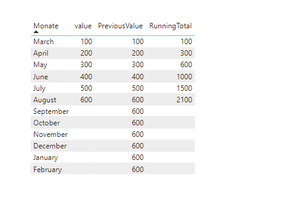As you can see running Total does not calculate the value of the column "PreviousValue".

For that I am using a measure, that helps to fill my missing value with the previous one. (Septemeber value == august value):

``````PreviousValue =
VAR _actualOrder = SELECTEDVALUE('Datetable'[Order])
VAR _mspOrder =
CALCULATE ( MAX('Table'[Order]), ALLSELECTED ('Table'))
VAR _maxdate =
CALCULATE ( MAX ( 'Table'[Data] ), ALLSELECTED ( 'Table' ) )
VAR _month =
MONTH ( _maxdate )
VAR _lastvalue =
CALCULATE (
SUM ( 'Table'[value] ),
FILTER (
ALLSELECTED ( 'Table' ),
YEAR ( 'Table'[Data] ) = YEAR ( _maxdate )
&& MONTH ( 'Table'[Data] ) = _month
)
)
RETURN
IF ( _actualOrder <= _mspOrder, SUM ( 'Table'[value] ), _lastvalue )``````

I already have a code for running total and it would be perfect if the solution is based on that code:
1.

``MinDate = Date(SELECTEDVALUE(Datetable[GJ]),3,1)``

2.

``````RunningTotal=
VAR minDate = [MinDate]

return
CALCULATE (
SUM('Table'[value]),
FILTER (
ALL ('Table'),
'Table'[Data] >= minDate
&&'Table'[Data] <= MAX ( 'Table'[Data])), FILTER (
ALL ('Table'),
'Table'[Order] <= MAX ( 'Table'[Order])))``````

I am sadly not able to add an file so I am giving you guys all the information here (Maybe someone can tell me how, so I can post it here)

Tables I am using:

OrderMonth (Running Total has to work between the first day of march and the last day of february (next year)

Because of that I created an order Table: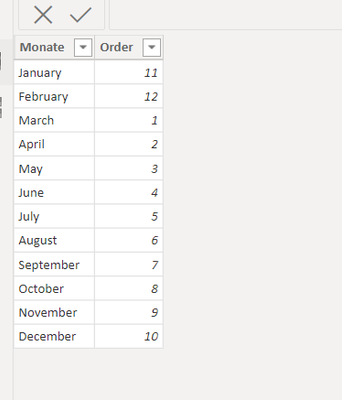Table:

(As you can see, there is not data for september, october etc.)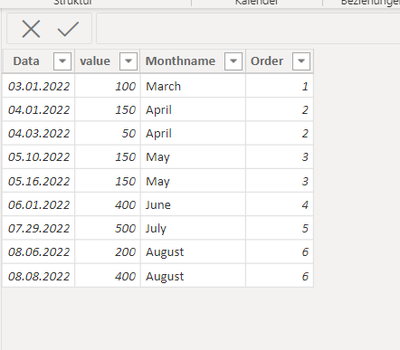Datetable: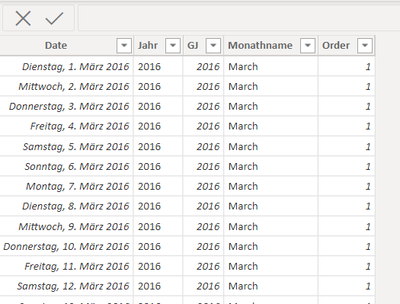I can give you to sourcecode:

``````let
Source = #date(2013, 1, 1),
#"add dates until today" = List.Dates(Source, Number.From(DateTime.LocalNow())+0- Number.From(Source), #duration(1,0,0,0)),
#"Converted to Table" = Table.FromList(#"add dates until today", Splitter.SplitByNothing(), null, null, ExtraValues.Error),
#"Renamed Column Date" = Table.RenameColumns(#"Converted to Table",{{"Column1", "Datum"}}),
#"Changed Type of Column Date" = Table.TransformColumnTypes(#"Renamed Column Date",{{"Datum", type date}}),
#"Hinzugefügte benutzerdefinierte Spalte3" = Table.AddColumn(#"Changed Type of Column Date", "Jahr", each Date.Year([Datum])),
#"Hinzugefügte benutzerdefinierte Spalte" = Table.AddColumn(#"Hinzugefügte benutzerdefinierte Spalte3", "GJ", each if [Datum] <> null then (if Date.Month([Datum])  then Date.Year([Datum])-1 else Date.Year([Datum])) else null),
#"Hinzugefügte benutzerdefinierte Spalte1" = Table.AddColumn(#"Hinzugefügte benutzerdefinierte Spalte", "Monatsname", each Date.MonthName([Datum])),
#"Geänderter Typ" = Table.TransformColumnTypes(#"Hinzugefügte benutzerdefinierte Spalte1",{{"GJ", Int64.Type}}),
#"Zusammengeführte Abfragen" = Table.NestedJoin(#"Geänderter Typ", {"Monatsname"}, OrderMonth, {"Monate"}, "OrderMonth", JoinKind.LeftOuter),
#"Erweiterte OrderMonth" = Table.ExpandTableColumn(#"Zusammengeführte Abfragen", "OrderMonth", {"Order"}, {"OrderMonth.Order"}),
#"Umbenannte Spalten" = Table.RenameColumns(#"Erweiterte OrderMonth",{{"OrderMonth.Order", "Order"}, {"Monatsname", "Monathname"}, {"Datum", "Date"}})
in
#"Umbenannte Spalten"``````

Relationshipmodel: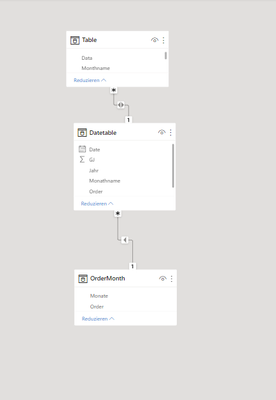1 ACCEPTED SOLUTIONCommunity Support

Hi, @anoonymous

You can try the following methods.

``````PreviousValue =
VAR _a = SUM('Table'[Value])
Var _b = CALCULATE(SUM('Table'[Value]),FILTER(ALL('Table'),[Order]=MAXX(ALL('Table'),[Order])))
return IF(ISBLANK(_a),_b,_a)````````RunningTotal = SUMX(FILTER(ALL(OrderMonth),[Order]<=SELECTEDVALUE(OrderMonth[Order])),[PreviousValue])``
``````RunningTotal 2 =
Var _N1=SUM('Table'[Value])
Var _N2=SUMX(FILTER(ALL(OrderMonth),[Order]<=SELECTEDVALUE(OrderMonth[Order])),[PreviousValue])
Var _N3=CALCULATE(SUM('Table'[Value]),ALL('Table'))
Return
IF(ISBLANK(_N1),_N3,_N2)``````Is this the result you expect?

Best Regards,

Community Support Team _Charlotte

If this post helps, then please consider Accept it as the solution to help the other members find it more quickly.

3 REPLIES 3Community Support

Hi, @anoonymous

You can try the following methods.

``````PreviousValue =
VAR _a = SUM('Table'[Value])
Var _b = CALCULATE(SUM('Table'[Value]),FILTER(ALL('Table'),[Order]=MAXX(ALL('Table'),[Order])))
return IF(ISBLANK(_a),_b,_a)````````RunningTotal = SUMX(FILTER(ALL(OrderMonth),[Order]<=SELECTEDVALUE(OrderMonth[Order])),[PreviousValue])``
``````RunningTotal 2 =
Var _N1=SUM('Table'[Value])
Var _N2=SUMX(FILTER(ALL(OrderMonth),[Order]<=SELECTEDVALUE(OrderMonth[Order])),[PreviousValue])
Var _N3=CALCULATE(SUM('Table'[Value]),ALL('Table'))
Return
IF(ISBLANK(_N1),_N3,_N2)``````Is this the result you expect?

Best Regards,

Community Support Team _Charlotte

If this post helps, then please consider Accept it as the solution to help the other members find it more quickly.Helper I

I know this is marked as solved but a problem came up.

As you can see some values don't have a "date" so previousMonth = the value where month is null and running total is starting right there and not march..

I hope I was able to make it clear...Helper I

Thank you so so so much!Announcements#### Exclusive opportunity for Women!

Join us for a free, hands-on Microsoft workshop led by women trainers for women where you will learn how to build a Dashboard in a Day!#### Power Platform Conference-Power BI and Fabric Sessions

Join us Oct 1 - 6 in Las Vegas for the Microsoft Power Platform Conference.Top Solution Authors
Top Kudoed Authors
Users online (1,833)